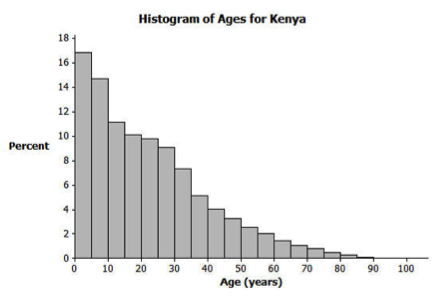# Descriptive Statistics

## Objective

Describe statistics. Represent data in frequency graphs and identify the center of a data set.

## Common Core Standards

### Core Standards

?

• HSS-ID.A.1 — Represent data with plots on the real number line (dot plots, histograms, and box plots).

• HSS-ID.A.2 — Use statistics appropriate to the shape of the data distribution to compare center (median, mean) and spread (interquartile range, standard deviation) of two or more different data sets.

• HSS-IC.A.1 — Understand statistics as a process for making inferences about population parameters based on a random sample from that population.

?

• 7.SP.A.1

• 7.SP.B.4

## Criteria for Success

?

1. Explain that statistics is the science of collecting and analyzing large amounts of information.
2. Explain descriptive statistics as summarizing the information collected.
3. Explain that inferential statistics is when you use information collected from a sample to make inferences about a population.
4. Describe the similarities and differences between the frequency graphs of bar graphs and histograms.

## Tips for Teachers

?

Students will need notes that allow them to organize their thinking around shape, center, and spread. Over this set of lessons, up through lesson 9, students will be using the terms shape, center and spread, and will need to parse how the two different measures of center (mean and median) relate to the two main measures of spread (standard deviation and interquartile range), through analysis of the shape (skew left, symmetrical, skew right). Students will also need to think about how the graphical representations of histograms and box plots relate to these concepts. Start the work to organize their thinking in this lesson and continue this through lesson 9.

## Anchor Problems

?

### Problem 1

After watching Why Statistics from the U.S. Census Bureau and Why You Need to Study Statistics from This is Statistics, what is statistics and how can it be applied?

### Problem 2

Below are two data representations. How are these representations similar? Different?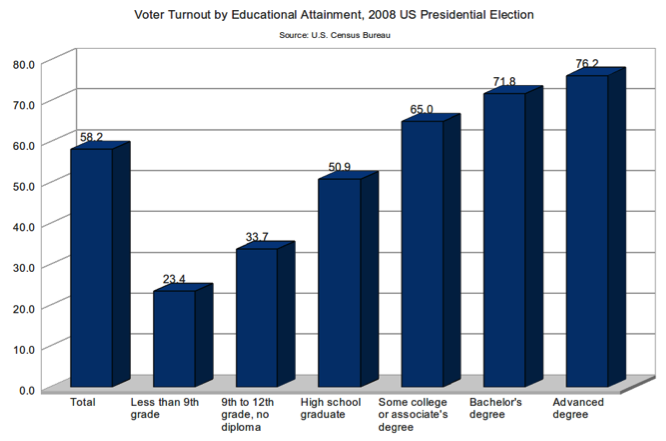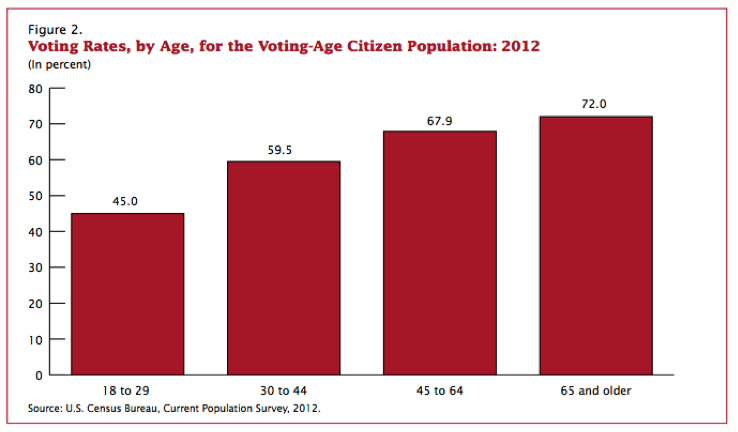### Problem 3

Aaron wanted to answer the question “How old are people in Boston?” He accessed this data from the US Census Bureau: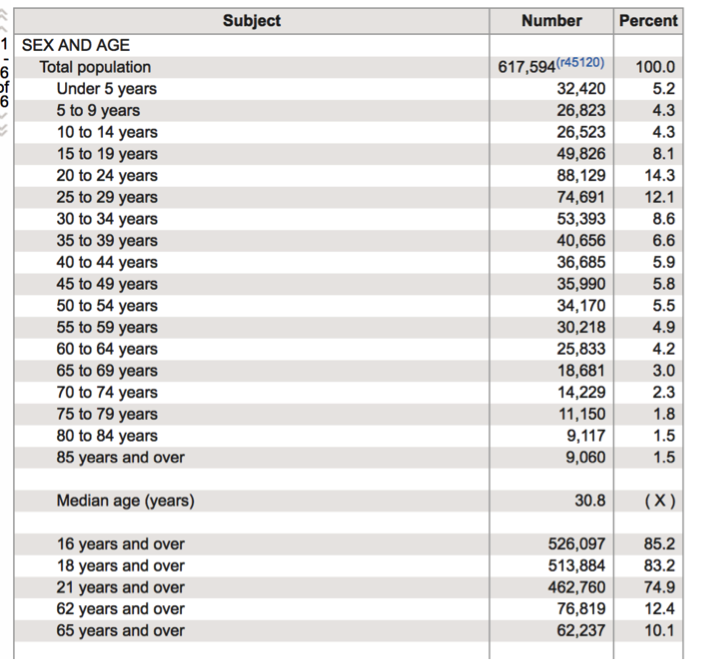Aaron looked at the data and was unsure of how to answer his question. He summarized the data from the table above into the histogram below.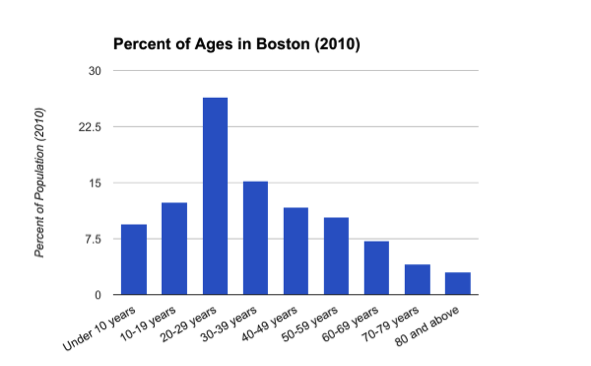How would you answer Aaron’s question based on the histogram of data?

#### References

U.S. Census Bureau US Census Bureau Fact Finder

## Problem Set

?

The following resources include problems and activities aligned to the objective of the lesson that can be used to create your own problem set.